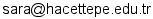# Announcements

1. Course page is on line (Oct.8)
2. A List of Bookstores where you can purchase the textbook is posted. (Oct. 8)
3. Homework 1 Questions posted. (Oct.12)
4. Homework 2 Questions posted. (Oct.26)
5. Homework 3 Questions posted. (Nov.4)
6. Homework 1 Answers posted. (Nov. 9)
7. Homework 2 Answers posted. (Nov.9)
8. Homework 3 Answers posted. (Nov.10)
9. Midterm Exam I Result posted. (Dec. 7)
10. Homework 4 part1, part2 Questions posted. (Dec. 19)
12. Homework 5 part 1 Questions and Answers posted. (Dec. 23)
13. Midterm Exam II Result posted. (Jan. 6)
14. Final Exam Schedule: Jan.26(Wed) 11:00 - 12:45. A2, D11. Coverage: ALL Chapters. Bring a calculator which can compute sq.root, e, power. No cell phones allowed. (Jan. 6)
15. Homework 5 part 2 Questions posted. (Jan. 6)
16. Homework 5 part2 Answers posted. (Jan.12)
17. Final Exam Result posted. (Jan.31)

# Personnel

Instructor: Shihomi Ara-Aksoy (Office: İİBF, İktisat Bölümü, Email:)

# Course Information

Schedule: Wednesdays, 9:00 - 11:45, D9 By Appointment The purpose of this course is to introduce basic concepts of statistics. Methods of describing data, probability, discrete and continuous random variables and their probability distributions will be discussed. Students are expected to learn the tools for describing and analyzing given data and to be acquainted with probability theory and probability distributions. The topics covered in this course will be a foundation for the courses such as Statistics II and Econometrics. Therefore, make sure to understand each concept clearly.

# Texts

• Paul Newbold, William Carlson and Betty Thorne, Statistics for Business and Economics,  Seventh edition, Pearson Education, 2010.

# Lecture Schedule

## Contents

### Week 1

(Oct. 6)

Topics:
• Why study statistics?

### Week 2

(Oct.13)

Topics:
• Using Graphs to Describe Data
• Ch. 1

### Week 3

(Oct. 20)

Topics:
• Using Numerical Measures to Describe Data (1)
• Ch. 2

### Week 4

(Oct. 27)

Topics:
• Using Numerical Measures to Describe Data (2)
• Ch. 2

### Week 5

(Nov. 3)

Topics:
• Elements of Chance: Probability Methods (1)
• Ch. 3

### Week 6

(Nov. 10)

Topics:
• Elements of Chance: Probability Methods (2)
• Ch. 3

(Nov.17)

No Class

(TBA)

1st Midterm Exam

### Week 9

(Dec. 1)

Topics:
• Discrete Probability Distributions (1)
• Ch. 4
Week 10
(Dec.8)
Topics:
• Discrete Probability Distributions (2)
• Ch. 4

### Week 11

(Dec. 15)

Topics:
• Discrete Probability Distributions (3)
• Ch. 4

### Week 12

(Dec. 22)

Topics:
• Continuous Probability Distributions (1)
• Ch. 5

2nd Midterm Exam

### Week 13

(Dec. 29)

Topics:
• Continuous Probability Distributions (2)
• Ch. 5

### Week 14

(Jan. 5)

Topics:
• Continuous Probability Distributions (3)
• Ch. 5

### Week 15

(Jan. 12)

Topics:
• Continuous Probability Distributions (4)
• Ch. 5

(TBA)

Final Exam

# Exams

• 1st Midterm exam: to be announced
• 2nd Midterm exam: to be announced
• Final exam date: to be announced